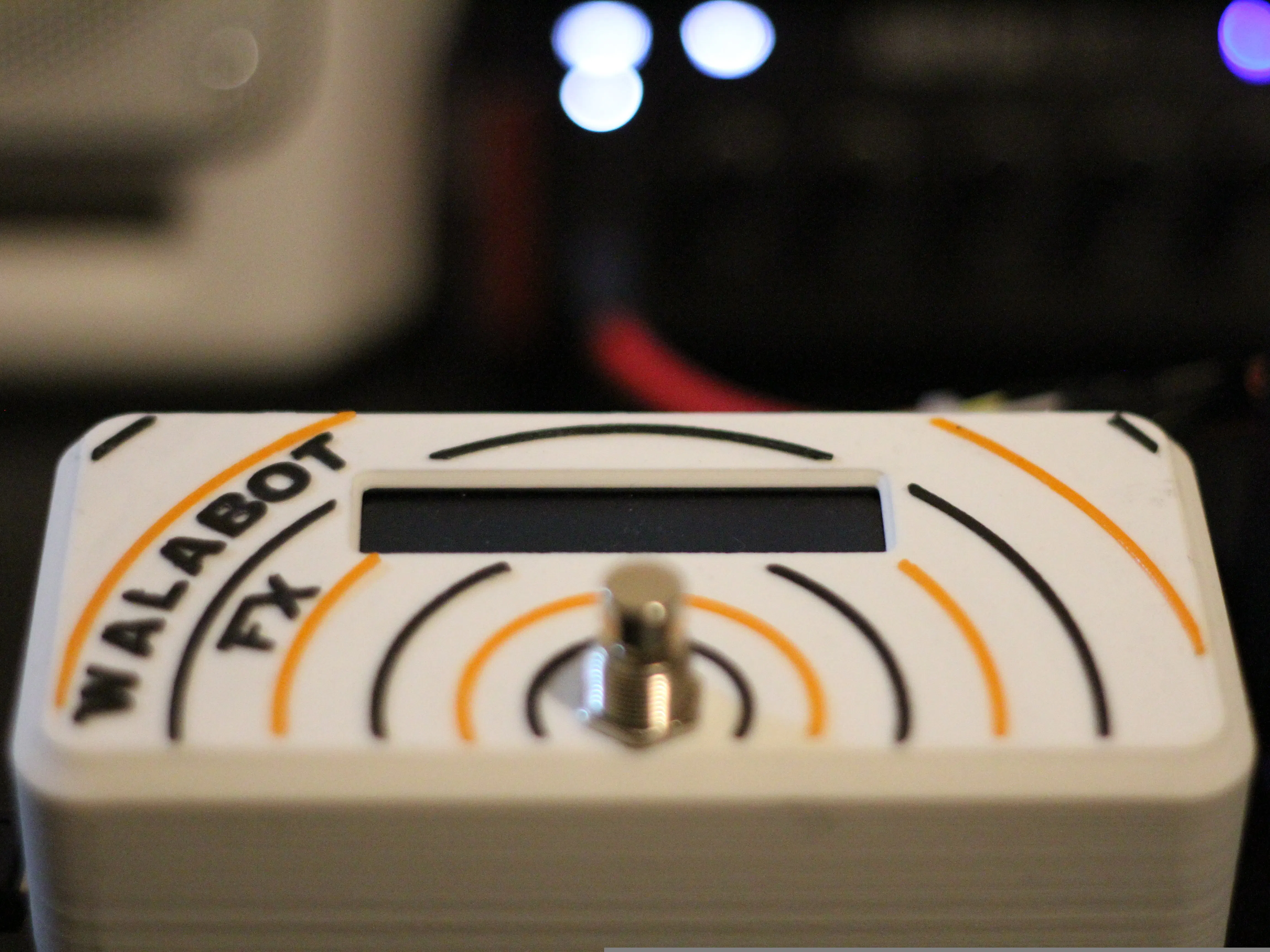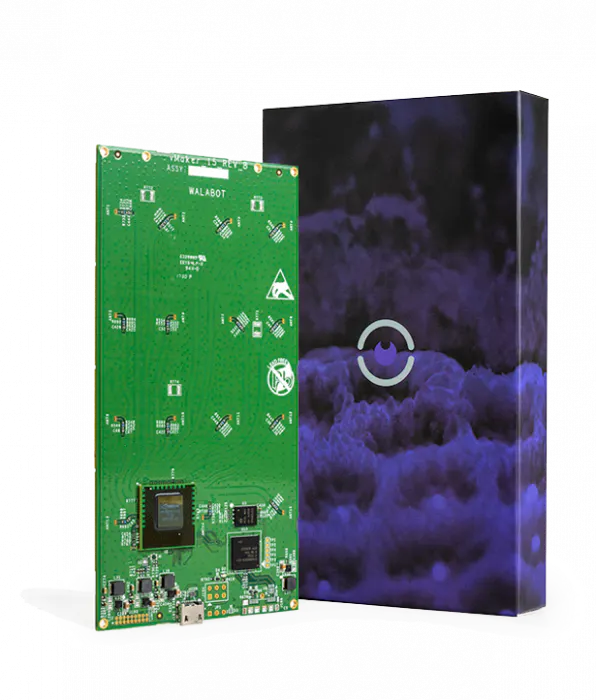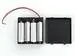# Walabot FX - Guitar Effect Control

Control your favourite guitar effect using nothing but awesome guitar poses.

IntermediateFull instructions provided2 days3,679## Things used in this project

### Hardware componentsWalabot Creator
×1Raspberry Pi 3 Model B
×1
 Sunfounder LCD1602
×1
 SunFounder PCA9685 16 Channel 12 Bit PWM Servo Driver for Arduino and Raspberry Pi
×1
 Servos (Tower Pro MG996R)
×39V Battery Clip
×14xAA battery holder
×1AA Batteries
×1Jumper wires (generic)
×2
 DPDT Latching Action Foot Switch
×1
 Korg SDD3000-PDL
×1

### Software apps and online servicesAutodesk Fusion 360Blynk

### Hand tools and fabrication machines3D Printer (generic)Soldering iron (generic)

## Custom parts and enclosures

### Walabot FX Servo Bracket

Use this to attach the servo to your effect

## Schematics

### Walabot FX Wiring Diagram

Use this with jumper wires to hook up your Walabot FX

## Code

### GuitarEffectCLI.py

Python
Use this to interface your walabot with servos
```from __future__ import print_function
from sys import platform
from os import system
from blynkapi import Blynk
import WalabotAPI
import time
import RPi.GPIO as GPIO

#set up GPIO using Board Numbering
GPIO.setmode(GPIO.BOARD)
GPIO.setup(18, GPIO.IN, pull_up_down = GPIO.PUD_UP)

#blynk auth token
auth_token = "auth_token_here"

# Import the PCA9685 module for servo control.

#import LCD module from location

# Initialise the PCA9685 using the default address (0x40).

# blynk objects
defaults = Blynk(auth_token, pin = "V9")
start_button = Blynk(auth_token, pin = "V3")
Rmax = Blynk(auth_token, pin = "V0")
Rmin = Blynk(auth_token, pin = "V1")
Rres = Blynk(auth_token, pin = "V2")

ThetaMax = Blynk(auth_token, pin = "V4")
ThetaRes = Blynk(auth_token, pin = "V5")

PhiMax = Blynk(auth_token, pin = "V6")
PhiRes = Blynk(auth_token, pin = "V7")

Threshold = Blynk(auth_token, pin = "V8")

ServoMin = Blynk(auth_token, pin = "V10")
ServoMax = Blynk(auth_token, pin = "V11")

def LCDsetup():
LCD1602.init(0x27, 1)   # init(slave address, background light)

def numMap(x, in_min, in_max, out_min, out_max):
""" used for mapping the walabot readings to servo position """
return int((x- in_min) * (out_max - out_min) / (in_max - in_min) + out_min)

# use this for rounding up the raw data to the assigned value
def myRound(x, base=2):
return int(base * round(float(x)/base))

#extracts the number form the returned blynk string
def numberExtract(val):
val = str(val)
return int(filter(str.isdigit, val))

# Set frequency to 60hz, good for servos.
pwm.set_pwm_freq(60)

# Configure min and max servo pulse lengths defaults
SERVO_MIN = 175 # Min pulse length out of 4096
SERVO_MAX = 575  # Max pulse length out of 4096

# walabot default values
R_MAX = 60
R_MIN = 20
R_RES = 5

THETA_MAX = 20
THETA_RES = 5

PHI_MAX = 20
PHI_RES = 5

THRESHOLD = 1

# variables for blynk switching
on = "[u'1']"

class Walabot:

def __init__(self):
self.wlbt = WalabotAPI
self.wlbt.Init()
self.wlbt.SetSettingsFolder()
self.isConnected = False
self.isTargets = False
self.distance = lambda t: (t.xPosCm**2+t.yPosCm**2+t.zPosCm**2) ** 0.5

def blynkConfig(self):
SERVO_MAX = ServoMax.get_val()
SERVO_MAX = numberExtract(SERVO_MAX)
print("Servo Max =", SERVO_MAX)

SERVO_MIN = ServoMin.get_val()
SERVO_MIN = numberExtract(SERVO_MIN)
print("Servo MIN =", SERVO_MIN)

R_MAX = Rmax.get_val()
R_MAX = numberExtract(R_MAX)
print("R max =", R_MAX)

R_MIN = Rmin.get_val()
R_MIN = numberExtract(R_MIN)
print("R Min =", R_MIN)

R_RES = Rres.get_val()
R_RES = numberExtract(R_RES)
print("R Res =", R_RES)

THETA_MAX = ThetaMax.get_val()
THETA_MAX = numberExtract(THETA_MAX)
print("Theta Max =", THETA_MAX)

THETA_RES = ThetaRes.get_val()
THETA_RES = numberExtract(THETA_RES)
print("Theta Res =", THETA_RES)

PHI_MAX = PhiMax.get_val()
PHI_MAX = numberExtract(PHI_MAX)
print("Phi Max =", PHI_MAX)

PHI_RES = PhiRes.get_val()
PHI_RES = numberExtract(PHI_RES)
print("Phi Res =", PHI_RES)

THRESHOLD = Threshold.get_val()
THRESHOLD = numberExtract(THRESHOLD)
print("Threshold =", THRESHOLD)

else: # if nothing from blynk app, load defaults
SERVO_MIN = 175 # Min pulse length out of 4096
SERVO_MAX = 575  # Max pulse length out of 4096

# walabot default values
R_MAX = 60
R_MIN = 20
R_RES = 5

THETA_MAX = 20
THETA_RES = 5

PHI_MAX = 20
PHI_RES = 5

THRESHOLD = 1

def connect(self):
try:
self.wlbt.ConnectAny()
self.isConnected = True
self.wlbt.SetProfile(self.wlbt.PROF_SENSOR)
#self.wlbt.SetProfile(self.wlbt.PROF_TRACKER)
#self.wlbt.SetDynamicImageFilter(self.wlbt.FILTER_TYPE_MTI)
self.wlbt.SetDynamicImageFilter(self.wlbt.FILTER_TYPE_NONE)
#self.wlbt.SetDynamicImageFilter(self.wlbt.FILTER_TYPE_DERIVATIVE)
self.wlbt.SetArenaTheta(-THETA_MAX, THETA_MAX, THETA_RES)
self.wlbt.SetArenaPhi(-PHI_MAX, PHI_MAX, PHI_RES)
self.wlbt.SetArenaR(R_MIN, R_MAX, R_RES)
self.wlbt.SetThreshold(THRESHOLD)

except self.wlbt.WalabotError as err:
if err.code != 19:  # 'WALABOT_INSTRUMENT_NOT_FOUND'
raise err

def start(self):
self.wlbt.Start()

def calibrate(self):
self.wlbt.StartCalibration()

def get_targets(self):
self.wlbt.Trigger()
#return self.wlbt.GetTrackerTargets()
return self.wlbt.GetSensorTargets()

def stop(self):
self.wlbt.Stop()

def disconnect(self):
self.wlbt.Disconnect()
self.wlbt.Clean()

def main():
flag = True
check = ""
LCDsetup()
while flag:
LCD1602.write(0, 0, 'Guitar        ')
LCD1602.write(0, 1, 'Effect Control')
time.sleep(2)
LCD1602.write(0, 0, 'Press Start to  ')
LCD1602.write(0, 1, 'begin           ')
time.sleep(2)
if (str(check) == on):
flag = False
else:
check = start_button.get_val()  #check for blynk start button press

if (GPIO.input(18) == 0): #check footswitch
flag = False

LCD1602.write(0, 0, "OK! let's Do it ")
LCD1602.write(0, 1, '                ')

wlbt = Walabot()
wlbt.blynkConfig()
wlbt.connect()
LCD1602.clear()
if not wlbt.isConnected:
LCD1602.write(0, 0, 'Not Connected ')
else:
LCD1602.write(0, 0, 'Connected     ')
time.sleep(2)
wlbt.start()
wlbt.calibrate()
LCD1602.write(0, 0, 'Calibrating.....')
time.sleep(3)
LCD1602.write(0, 0, 'Starting Walabot')

appcheck = start_button.app_status()

flag = True # reset flag for main prog

while flag: # used to put effect in standby (effectively)
if (appcheck == True):
if (str(check) != on):
if (GPIO.input(18) != 0): #check footswitch
flag = False
else:
check = start_button.get_val()  #check for start button press
appcheck = start_button.app_status()

else:
if (GPIO.input(18) != 0): #check footswitch
flag = False

xval = 0
yval = 0
zval = 0

average = 1
delayTime = 0

targets = wlbt.get_targets()

if len(targets) > 0:
for j in range(average):

targets = wlbt.get_targets()
if len(targets) > 0:

print(len(targets))
targets = targets

print(str(targets.xPosCm))
xval += int(targets.xPosCm)
yval += int(targets.yPosCm)
zval += int(targets.zPosCm)
time.sleep(delayTime)
else:
print("no targets")

xval = xval/average

xval = numMap(xval, -60, 60, SERVO_MIN, SERVO_MAX)
xval = myRound(xval)
if xval < SERVO_MIN: # protect the servo
xval = SERVO_MIN
if xval > SERVO_MAX:
xval = SERVO_MAX
LCD1602.write(0, 0, 'x=' + str(xval) + '           ')
pwm.set_pwm(0, 0, xval)

yval = yval/average

yval = numMap(yval, -60, 60, SERVO_MIN, SERVO_MAX)
yval = myRound(yval)
if yval < SERVO_MIN: # protect the servo
yval = SERVO_MIN
if yval > SERVO_MAX:
yval = SERVO_MAX
LCD1602.write(0, 1, 'y=' + str(yval))
pwm.set_pwm(1, 0, yval)

zval = zval/average

zval = numMap(zval, R_MIN, R_MAX, SERVO_MIN, SERVO_MAX)
zval = myRound(zval)
if zval < SERVO_MIN: # protect the servo
zval = SERVO_MIN
if zval > SERVO_MAX:
zval = SERVO_MAX
LCD1602.write(8, 1, 'z=' + str(zval))
pwm.set_pwm(2, 0, zval)

else:
print("no targets")
LCD1602.write(0, 0, "Shutting Down   ")
LCD1602.write(0, 1, 'The Walabot     ')
time.sleep(3)
wlbt.stop()
wlbt.disconnect()

if __name__ == '__main__':
while True:
main()
```

### guitareffect.sh

Powershell
use this to start your python code
```#!/bin/sh
cd /home/pi
sudo python GuitarEffectCLI.py
```

### rc.local

Powershell
```#!/bin/sh -e
#
# rc.local
#
# This script is executed at the end of each multiuser runlevel.
# Make sure that the script will "exit 0" on success or any other
# value on error.
#
# In order to enable or disable this script just change the execution
# bits.
#
# By default this script does nothing.

_IP=\$(hostname -I) || true
if [ "\$_IP" ]; then
printf "My IP address is %s\n" "\$_IP"
fi

./blynk-library/linux/blynk --token="you token goes here" &
sleep 10
sudo /home/pi/guitareffect.sh &
exit 0
```

### LCD Python Lib

Use this for the Sunfounder LCD

## Credits

### Dave Clarke

14 projects • 82 followers
Industrial Designer, Maker of Things, Lover of Phi and all around nice guy